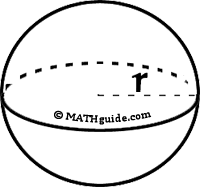MATHguide's Surface Area of Spheres Quizmaster
Review the Lesson | MATHguide homepage Updated July 21st, 2021

 When the diameter = 14 units, calculate the information below. Use 3.14 as an approximation for π and round all answers to the nearest tenth. What is the radius? r = unitsWhat is the circumference? C = units What is the surface area? TSA = units2